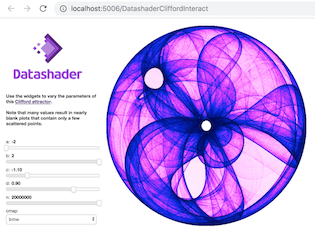# Clifford panel¶## Clifford attractors with Datashader, Panel, and interact¶

Clifford attractors are a type of iterative equation that traces the path of a particle through a 2D space using functions of sine and cosine terms that make interesting "attractor" patterns (covering only some portions of the possible space, in certain shapes).

Here we use Numpy and Pandas to calculate a dataframe consisting of millions of such locations, using Numba to make generating them 50X faster than bare Python. We'll then plot the results as a static image using Datashader, which renders arbitrarily large data into fixed-sized images.

If you can have a live Python process running (not just a static webpage or anaconda.org notebook viewer), we'll also show you how to make an interactive app for exploring parameter values, and a standalone dashboard suitable for sharing widely. Before you run the notebook or server, you'll need to set up a conda environment and run conda install -c pyviz datashader panel.

In :
import numpy as np, pandas as pd
from numba import jit

@jit(nopython=True)
def clifford_trajectory(a, b, c, d, x0, y0, n):
xs, ys = np.zeros(n), np.zeros(n)
xs, ys = x0, y0
for i in np.arange(n-1):
xs[i+1] = np.sin(a * ys[i]) + c * np.cos(a * xs[i])
ys[i+1] = np.sin(b * xs[i]) + d * np.cos(b * ys[i])
return xs, ys


We can visualize the resulting dataframe using Datashader, with colormaps from Colorcet:

In :
import datashader as ds
from datashader import transfer_functions as tf

from colorcet import palette_n
ps ={k:p[::-1] for k,p in palette_n.items()}

import panel as pn
pn.extension()

def clifford_plot(a=1.9, b=1.9, c=1.9, d=0.8, n=1000000, colormap=ps['kbc']):
cvs = ds.Canvas(plot_width=600, plot_height=600)
xs, ys = clifford_trajectory(a, b, c, d, 0, 0, n)
agg = cvs.points(pd.DataFrame({'x':xs, 'y':ys}), 'x', 'y')